### Home > PC3 > Chapter 8 > Lesson 8.2.2 > Problem8-73

8-73.
1. Given θ =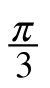, what is the exact value of each of the following trigonometric expressions? Homework Help ✎

1. sec(θ)

2. csc(θ)

3. tan(θ)

4. cot(θ)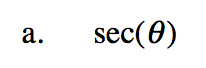$\frac{1}{\cos\left(\frac{\pi}{3}\right)}$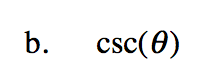$\frac{1}{\sin\left(\frac{\pi}{3}\right)}$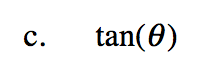$\frac{\sin\left(\frac{\pi}{3}\right)}{\cos\left(\frac{\pi}{3}\right)}$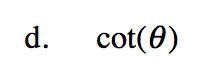$\frac{1}{\tan\left(\frac{\pi}{3}\right)}$